# Freeze-out radii extracted from three-pion cumulants in pp, p-Pb and Pb-Pb collisions at the LHC

In high-energy collisions, the spatio-temporal size of the particle production region can be measured using the Bose-Einstein correlations of identical bosons at low relative momentum. The source radii are typically extracted using two-pion correlations, and characterize the system at the last stage of interaction, called kinetic freeze-out. In low-multiplicity collisions, unlike in high-multiplicity collisions, two-pion correlations are substantially altered by background correlations, e.g. mini-jets. Such correlations can be suppressed using three-pion cumulant correlations. We present the first measurements of the size of the system at freeze-out extracted from three-pion cumulant correlations in pp, p-Pb and Pb-Pb collisions at the LHC with ALICE. At similar multiplicity, the invariant radii extracted in p-Pb collisions are found to be 5-15% larger than those in pp, while those in Pb-Pb are 35-55% larger than those in p-Pb. Our measurements disfavor models which incorporate substantially stronger collective expansion in p-Pb as compared to pp collisions at similar multiplicity.

Figures

## Figure 1

 Demonstration of the removal of the $K^0_s$ decay from three-pion cumulants. Mixed-charge three-pion correlations are projected against the relative momentum of a mixed-charge pair ($q_{31}^{\pm\mp}$). The $K^0_s$ decay into a $\pi^{+}+\pi^{-}$ pair is visible as expected around 0.4 GeV/$c$. The FSI enhancement of the mixed-charge pair 31" is also visible at low $q_{31}^{\pm\mp}$. FSI corrections are not applied. Systematic uncertainties are shown by shaded boxes.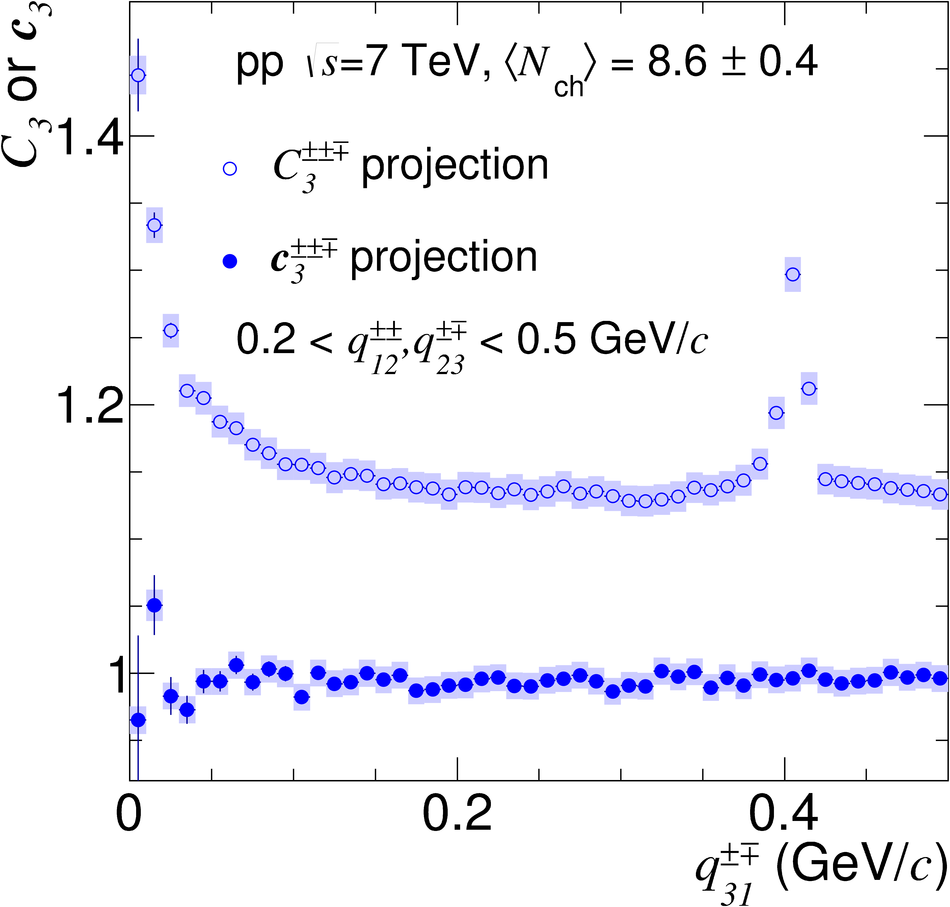## Figure 2

 Three-pion correlation functions versus $Q_3$ for $0.16< K_{\rm{T,3}} < 0.3$ GeV/$c$ in pp, p-Pb and Pb-Pb collision data compared to PYTHIA, DPMJET and HIJING generator-level calculations. Top panels are for same-charge triplets, while bottom panels are for mixed-charge triplets. Two points at low $Q_3$ with large statistical uncertainties are not shown for the pp same-charge correlation function.## Figure 3

 Two- and three-pion Gaussian fit parameters versus $\langle{N_{\rm ch}}\rangle$ in pp, p-Pb and Pb-Pb collision systems for low and high $\kT$ and $K_{\rm{T,3}}$ intervals. Top panels show the Gaussian radii $R^{\rm G}_{\rm inv}$ and $R^{\rm G}_{\rm inv,3}$ and bottom panels show the effective Gaussian intercept parameters $\lambda^{\rm G}_{\rm e}$ and $\lambda^{\rm G}_{\rm e,3}$. The systematic uncertainties are dominated by fit range variations and are shown by bounding/dashed lines and shaded boxes for two- and three-particle parameters, respectively. The dashed and dash-dotted lines represent the chaotic limits for $\lambda^{\rm G}_{\rm e}$ and $\lambda^{\rm G}_{\rm e,3}$, respectively.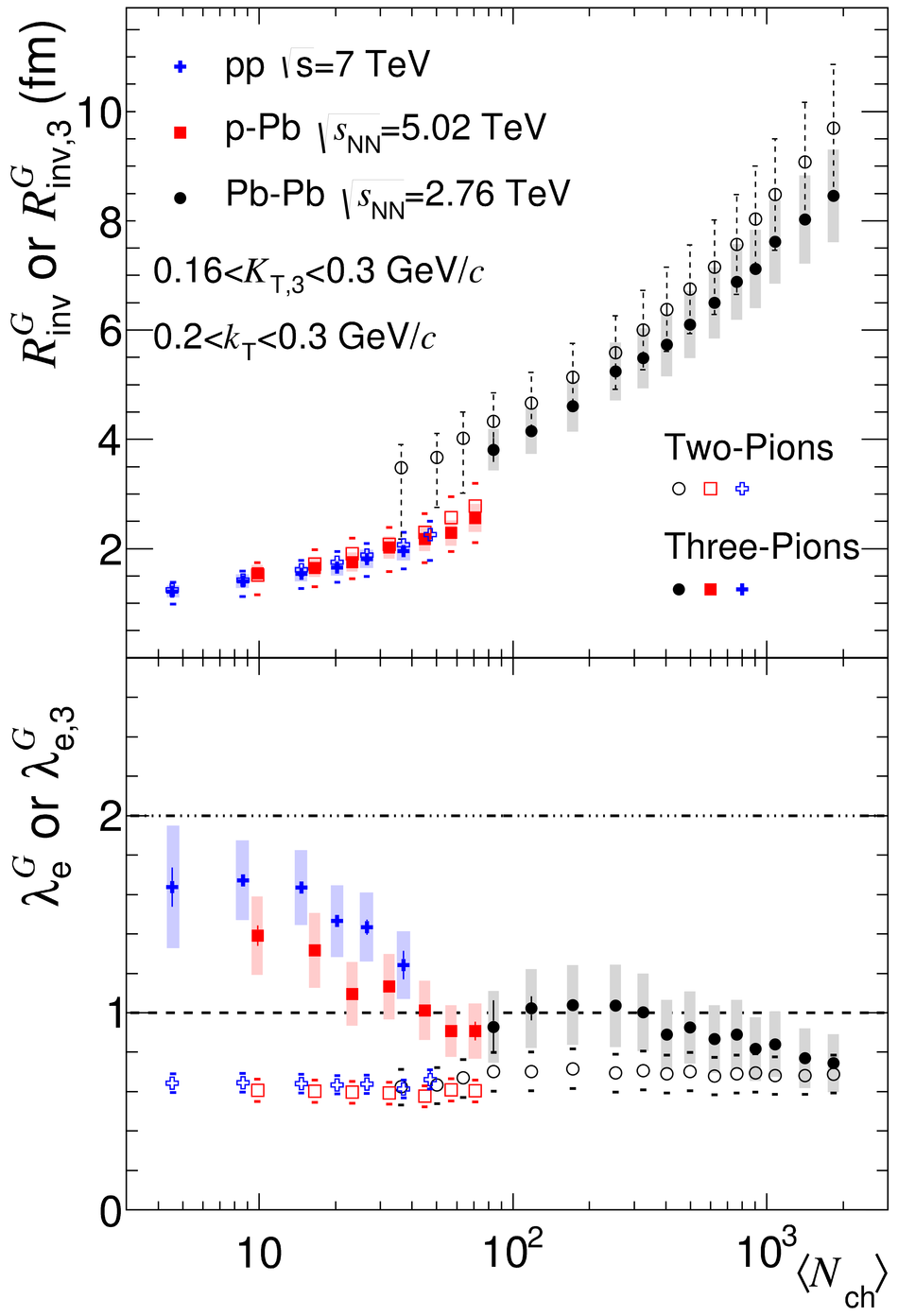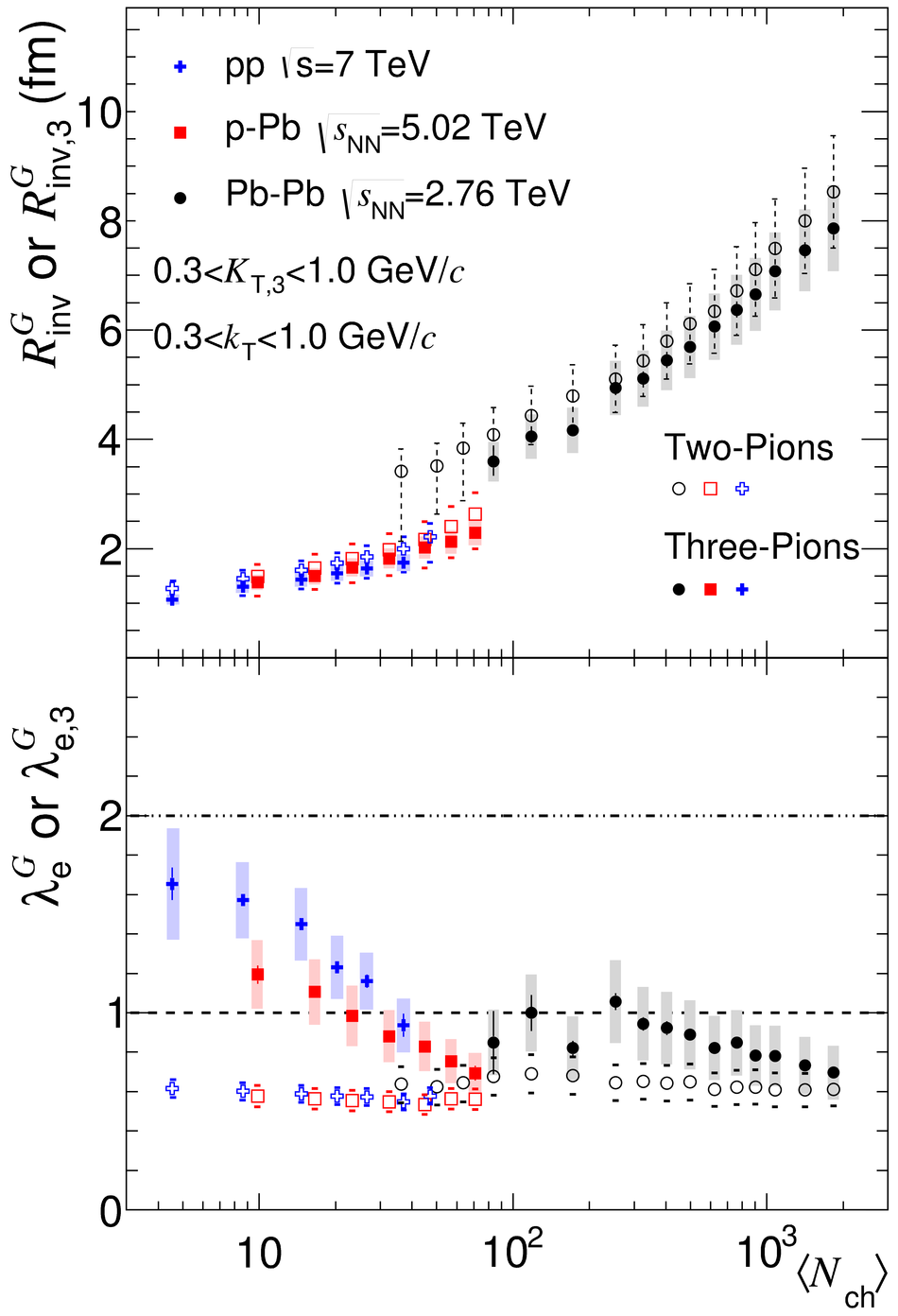## Figure 4

 Two- and three-pion Edgeworth fit parameters versus $\langle{N_{\rm ch}}\rangle$ in pp, p-Pb and Pb-Pb collision systems for low and high $\kT$ and $K_{\rm{T,3}}$ intervals. Top panels show the Edge-worth radii $R^{E_{\rm w}}_{\rm inv}$ and $R^{E_{\rm w}}_{\rm inv,3}$ and bottom panels show the effective intercept parameters $\lambda^{E_{\rm w}}_{\rm e}$ and $\lambda^{E_{\rm w}}_{\rm e,3}$. As described in the text, $\kappa_3$ and $\kappa_4$ are fixed to 0.1 and 0.5, respectively. Same details as for Fig.3.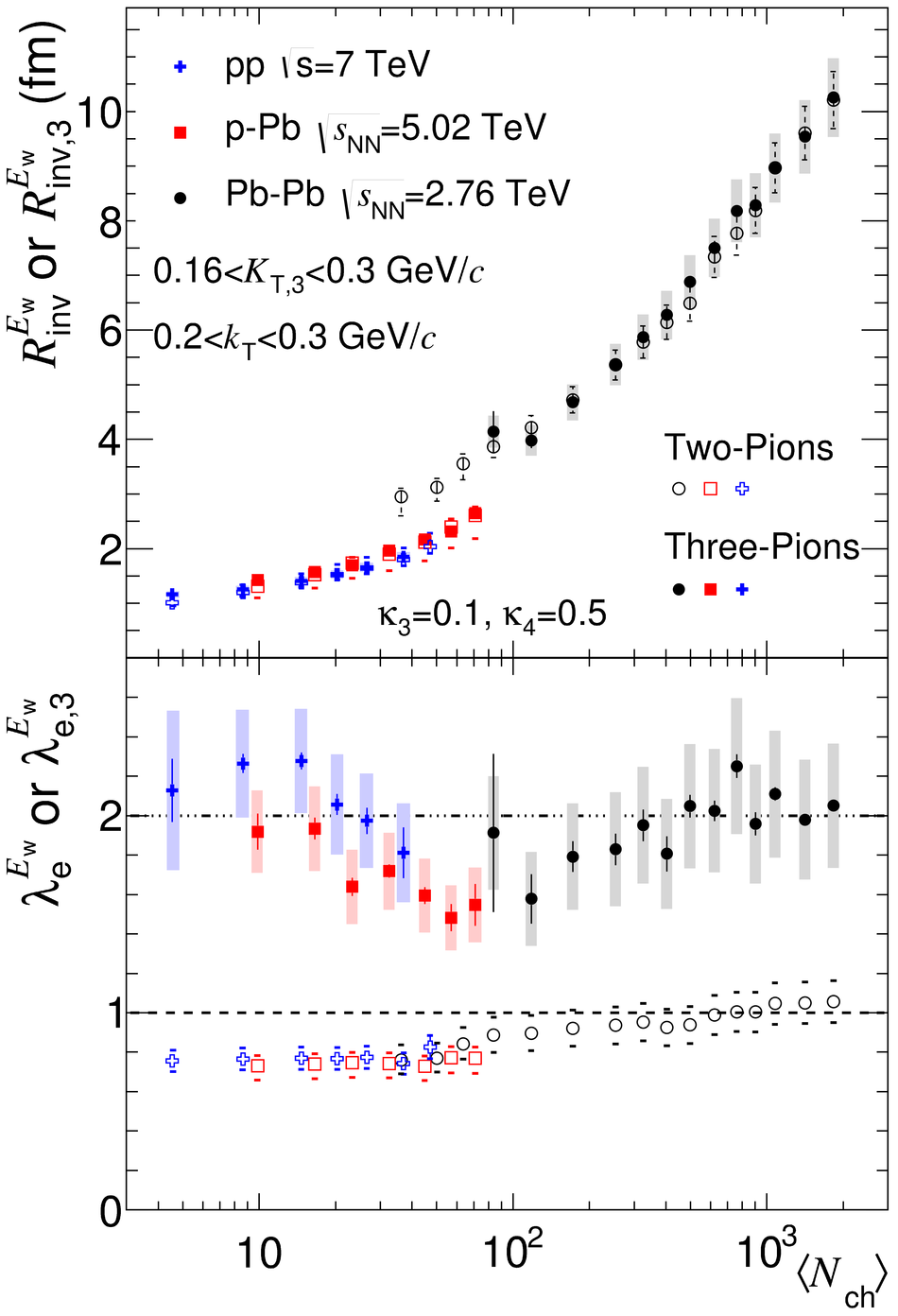## Figure 5

 Two- and three-pion Exponential fit parameters versus $\langle{N_{\rm ch}}\rangle$ in pp, p-Pb and Pb-Pb collision systems for low and high $\kT$ and $K_{\rm{T,3}}$ intervals. Top panels show the Exponential radii $R^{\rm Exp}_{\rm inv}$ and $R^{\rm Exp}_{\rm inv,3}$ scaled down by $\sqrt{\pi}$ and bottom panels show the effective intercept parameters $\lambda^{\rm Exp}_{\rm e}$ and $\lambda^{\rm Exp}_{\rm e,3}$. Same details as for Fig.3.## Figure 6

 Comparisons of same-charge three-pion cumulant correlation functions at similar multiplicity for $0.16< K_{\rm {T,3}}< 0.3$ GeV/$c$. Three points at low $Q_3$ with large statistical uncertainties are not shown in the left panel.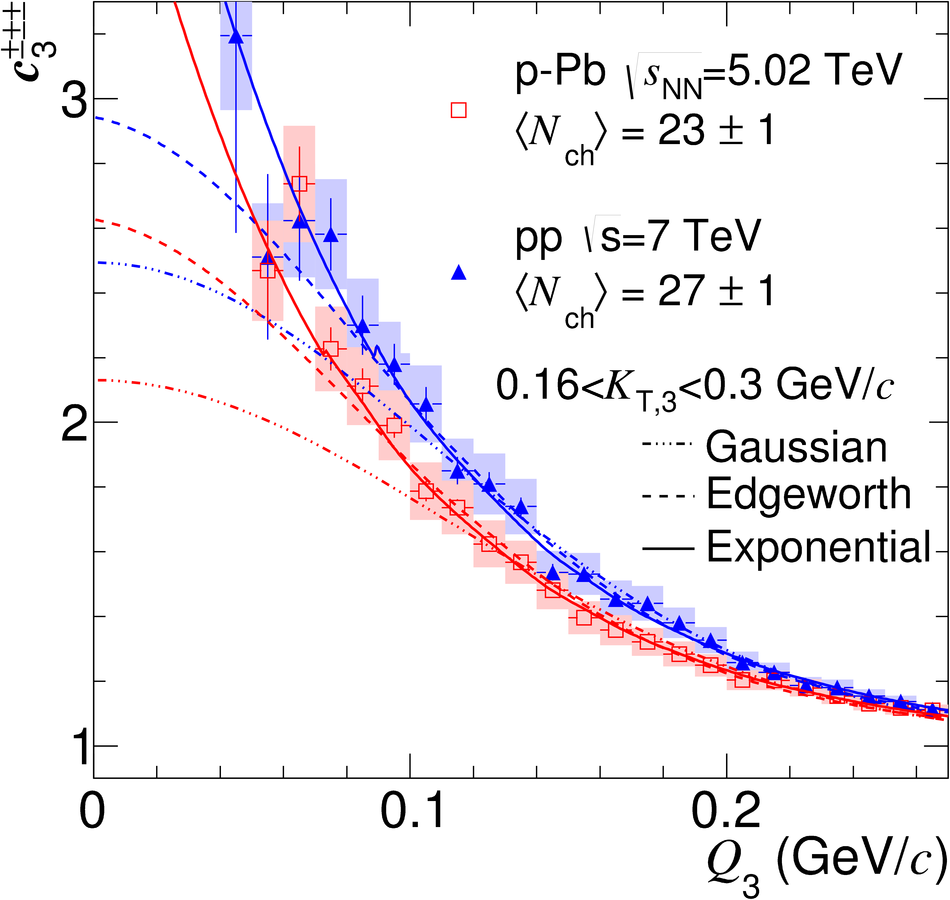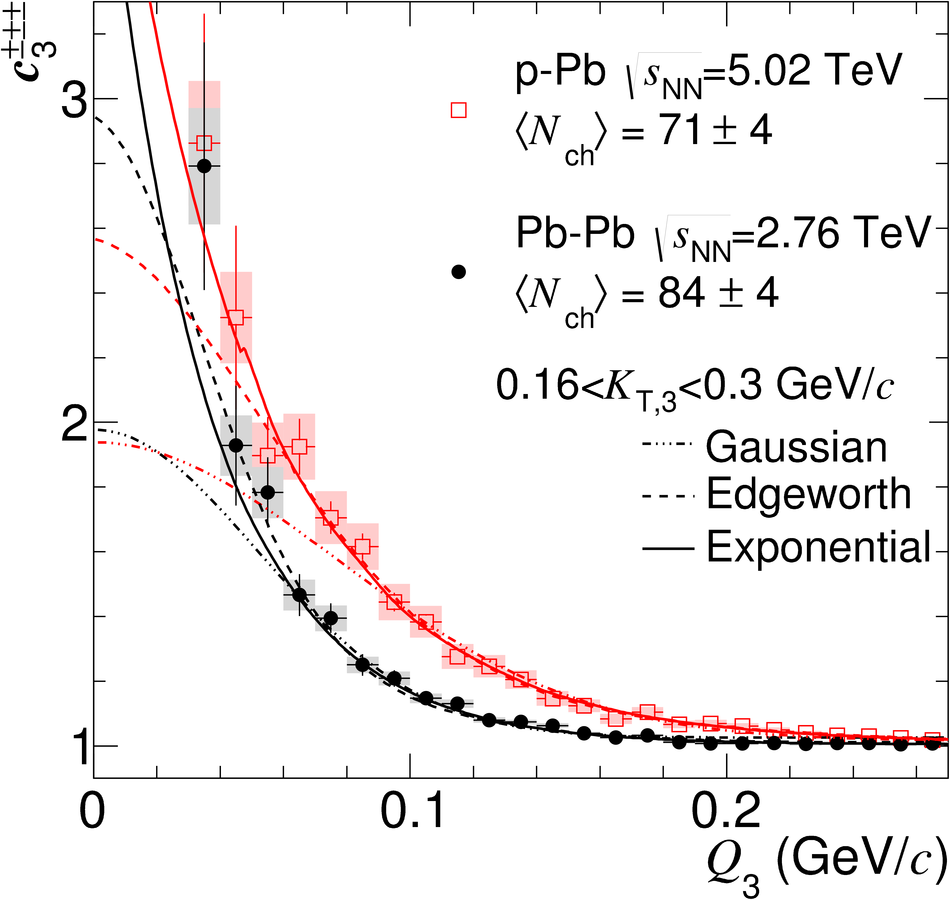## Figure 1

 Two- and three-pion Edgeworth fit parameters versus $\langle{N_{\rm ch}}\rangle^{1/3}$ in pp, p-Pb and Pb-Pb collision systems for low and high $\kT$ and $K_{\rm {T,3}}$ intervals. Top panels show the Edgeworth radii $R^{E_{\rm w}}_{\rm inv}$ and $R^{E_{\rm w}}_{\rm inv,3}$ and bottom panels show the effective intercept parameters $\lambda^{E_{\rm w}}_{\rm e}$ and $\lambda^{E_{\rm w}}_{\rm e,3}$. As described in the text, $\kappa_3$ and $\kappa_4$ are fixed to 0.1 and 0.5, respectively. The systematic uncertainties are dominated by fit-range variations and are shown by bounding lines and shaded boxes for two- and three-particle parameters, respectively. The dashed and dash-dotted lines represent the chaotic limits for $\lambda^{E_{\rm w}}_{\rm e}$ and $\lambda^{E_{\rm w}}_{\rm e,3}$, respectively. The low $\kT$ and $K_{\rm {T,3}}$ linear fit parameters for pp, p-Pb, and Pb-Pb are $0.436 + 0.403N_{\rm ch}^{1/3}$, $0.082 + 0.586N_{\rm ch}^{1/3}$, and $0.045 + 0.843N_{\rm ch}^{1/3}$, respectively, while for high $\kT$ and $K_{\rm {T,3}}$ they are $0.332 + 0.405N_{\rm ch}^{1/3}$, $-0.054 + 0.585N_{\rm ch}^{1/3}$, $0.049 + 0.772N_{\rm ch}^{1/3}$, respectively. For pp and p-Pb three-pion, while for Pb-Pb two-pion results are fit.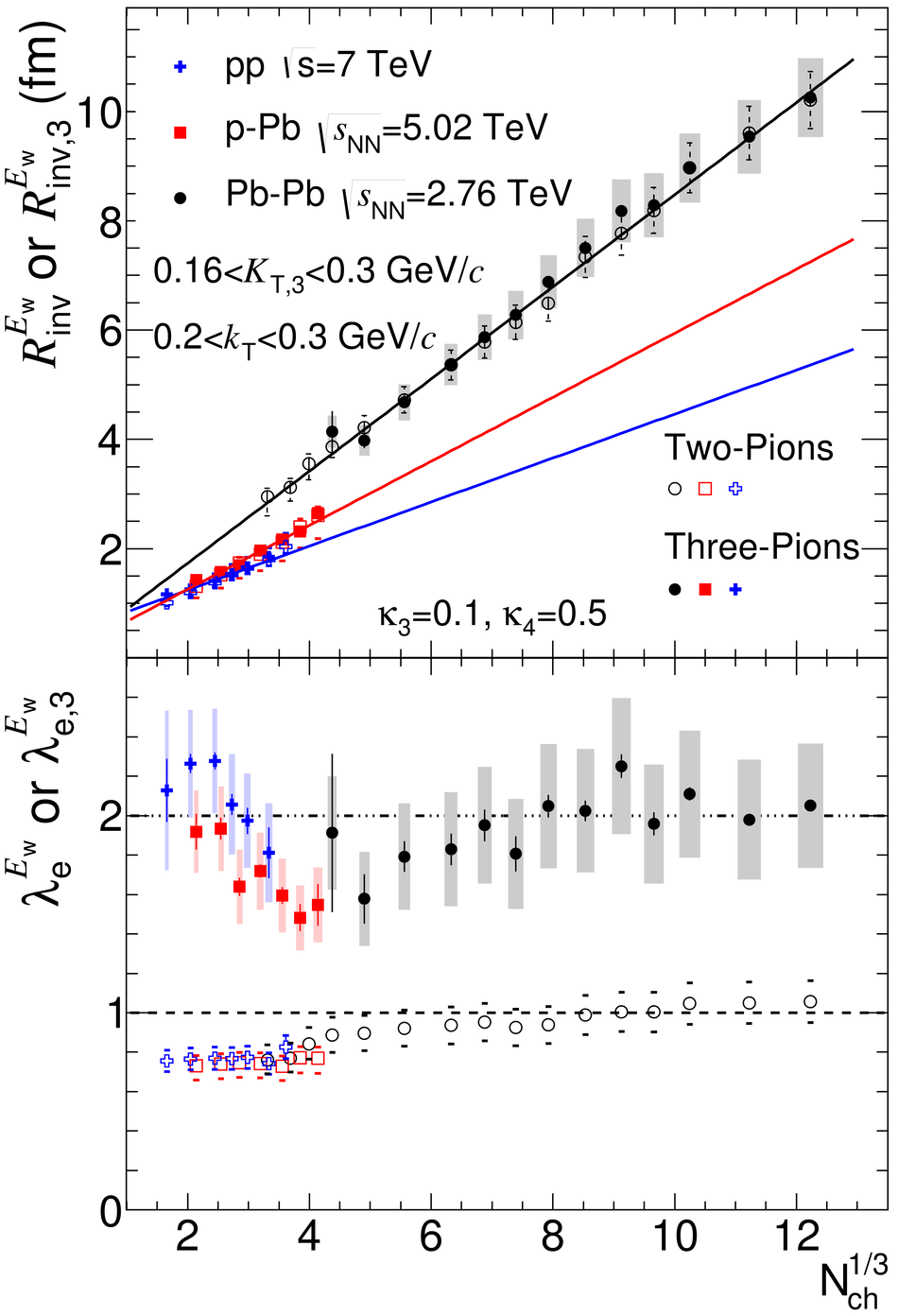## Figure 2

 The ratio of three-pion Edgeworth radii in p-Pb over the linear fit in pp collisions, and the ratio of two-pion Edgeworth radii in Pb-Pb over the linear fit in p-Pb collisions versus $\langle{N_{\rm ch}}\rangle^{1/3}$ for low and high $\kT$ and $K_{\rm {T,3}}$ intervals. Errors are statistical, since systematic errors largely cancel in the ratio.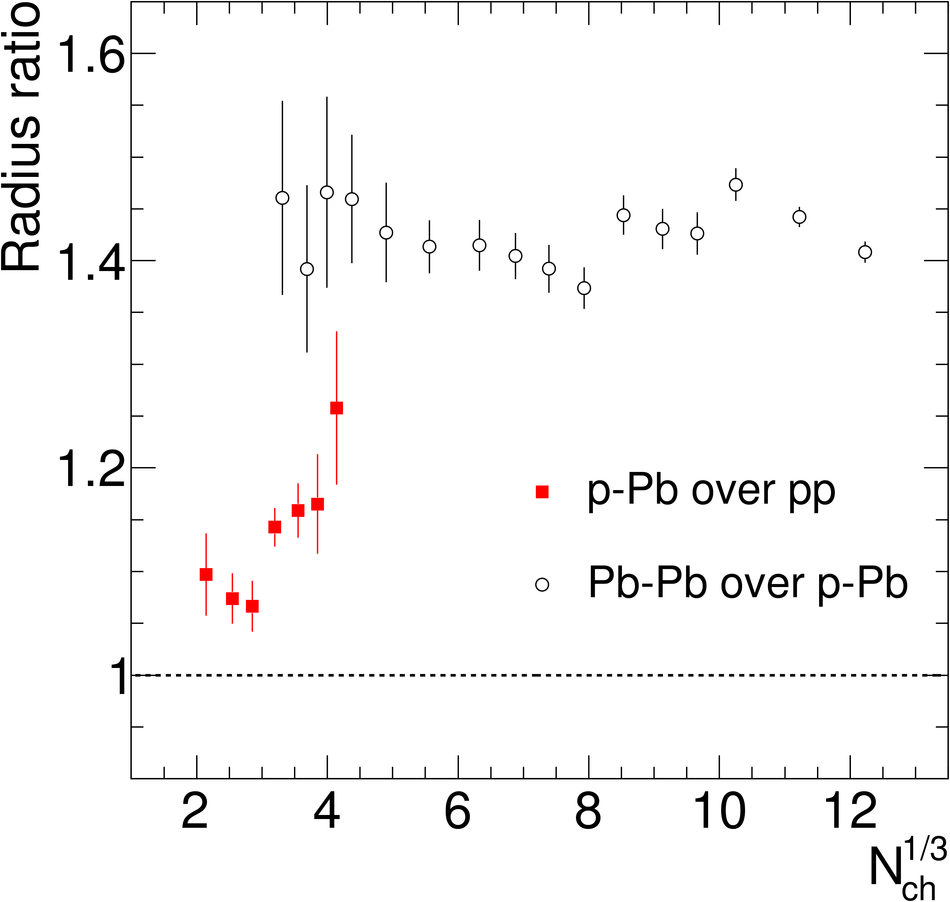## Figure 3

 For illustration: Two-pion correlations versus $q$ for $0.2< \kT< 0.3$ GeV/$c$ and three-pion cumulant functions versus $Q_3$ for $0.16< K_{\rm {T,3}} < 0.3$ GeV/$c$ with extended range. Final-state interactions have not been removed. Only statistical errors are shown.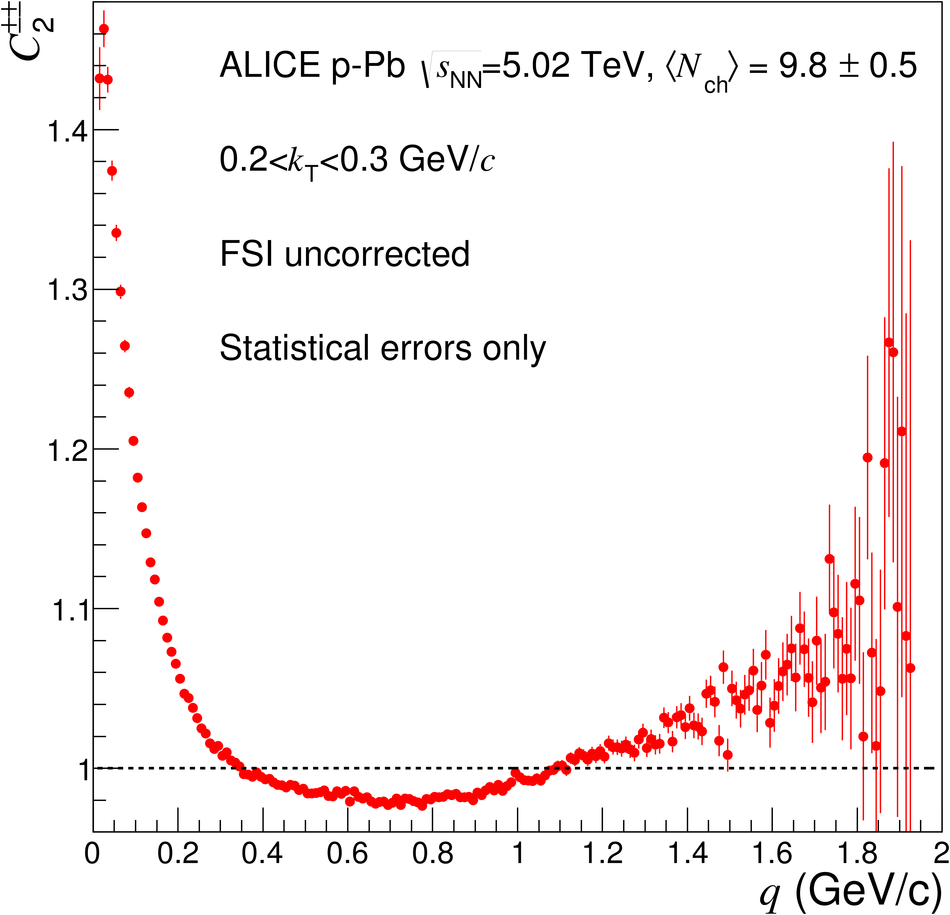## Figure 4

 Three-pion cumulant correlation functions for three sample multiplicity intervals in pp, p-Pb, and Pb-Pb. This figure demonstrates the span of 3-pion Bose-Einstein cumulants for collisions at the LHC.